Webucator's Free Java Tutorial

# Lesson: Arrays

Welcome to our free Java tutorial. This tutorial is based on Webucator's Introduction to Java Training course.

In this lesson, you will learn what arrays are, and how to use them.

Lesson Goals

• Understand how arrays are implemented in Java.
• Declare, instantiate, populate, and use arrays of primitives.
• Use arrays of objects.
• Use multi-dimensional arrays.

## Defining and Declaring Arrays

An array stores a group of data items all of the same type. An array is an object.

• An array variable does not actually store the array - it is a reference variable that points to an array object.
• Declaring the array variable does not create an array object instance; it merely creates the reference variable - the array object must be instantiated separately.
• Once instantiated, the array object contains a block of memory locations for the individual elements.
• If the individual elements are not explicitly initialized, they will be set to zero.
• Arrays can be created with a size that is determined dynamically (but once created, the size is fixed).

Declare an array variable by specifying the type of data to be stored, followed by square brackets `[]`.

## Syntax

`               dataType[] variableName;`

You can read the `[]` as the word "array".

To declare a variable for an array of integers:

`int[] nums;`

...which you can read as "`int` array `nums`".

To declare a variable for an array of `String` objects:

`String[] names;`

...which you can read as "`String` array `names`" - the array holds `String` references.

You may also put the brackets after the variable name (as in C/C++), but that is less clearly related to how Java actually works.

`int nums[]; // not recommended, but legal`

But, that syntax does allow the following, which is legal, but seems like a bad practice.

`int nums[], i, j; // declares one array and two single int values`

## Instantiating Arrays

Instantiate an array object using `new`, the data type, and an array size in square brackets

```int[] nums;
nums = new int;```

The second line constructs a new array object with 10 integer elements, all initialized to 0, and stores the reference into `nums`.

```int[] moreNums;
int size = 7;
moreNums = new int[size];```

You can declare and instantiate all at once:

`String[] names = new String;`

The elements of the array, `String` references, are initialized to `null`.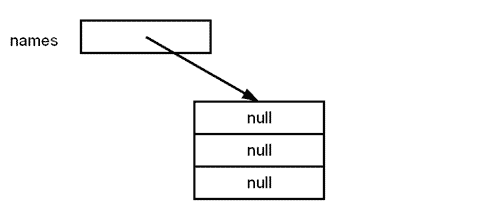As objects, arrays also have a useful property: `length`:

• In the above example, `names.length` would be 3.
• The property is fixed (i.e., it is read-only).

You can reassign a new array to an existing variable:

```int[] nums;
nums = new int;
nums = new int;```

The original ten-element array is no longer referenced by `nums`, since it now points to the new, larger array.

## Initializing Arrays

An array can be initialized when it is created

The notation looks like this:

`String[] names = { "Joe", "Jane", "Herkimer" };`

or

`String[] names = new String[] {"Joe", "Jane", "Herkimer" };`

This automatically creates an array of length 3, because there were 3 items supplied.

`int[] nums = new int[] { 2, 4, 6, 8, 10, 12 };`

This array will have a length of 6.

If a new array is being assigned to an existing variable, you cannot use the shorter variant, you must use the `new` keyword and the data type:

```String[] names;
names = new String[] {"Joe", "Jane", "Herkimer" };```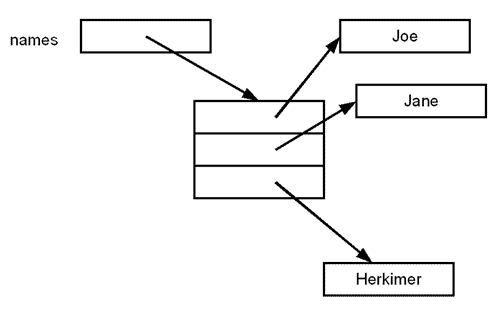For arrays of other types of objects:

```Book[] titles;
titles = new Book[] {
new Book(5011,"Fishing Explained"),
new Book(1234, "Help is on the Way")
};```

If we were to run the code above, we would end up with an array named "`titles`" each of whose two elements is an object of type `Book`.

## Working with Arrays

Array elements are accessed through the array reference, by their array index:

• The index is the element number, placed within brackets.
• Elements are numbered from 0 to one less than the specified size.
`String[] names = new String;`

The valid elements are 0, 1, and 2, as in:

```names = "Sam";
names = "Sue";
names = "Mary";```

You could access array elements in a for loop with:

`for (int i = 0; i < 3; i++) System.out.println(names[i]);`

Or, better programming practice would be to use the `length` property:

`for (int i = 0; i < names.length; i++) System.out.println(names[i]);`

The compiler does not check to ensure that you stay within the bounds of the array, but the JVM does check at runtime - if you try to exceed the bounds of the array, an exception will occur.

Note that a zero-length array is valid:

```Book[] titles = new Book[] { };
Book[] moreTitles = new Book;```

You might create a zero-length array as the return value from a method typed as returning an array, when there are no items to return (as opposed to returning `null`).

## Code Sample:

Java-Arrays/Demos/Arrays1.java
```import java.util.*;
public class Arrays1 {
public static void main(String[] args) {
Random r = new Random();
int[] nums = new int;
for (int i = 0; i < nums.length; i++) {
nums[i] = r.nextInt(100);
}
System.out.println("Element 7 is: " + nums);
String[] names = new String;
names = "Joe";
names = "Jane";
names = "Herkimer";
for (int i = 0; i < names.length; i++) {
System.out.println(names[i]);
}
//this line should throw an exception
System.out.println(names);
}
}```

### Code Explanation

In the demo above, line 18 throws an exception: since array `names` has three elements, trying to access `names` gives an error.

## Enhanced `for` Loops - the For-Each Loop

In the last lesson we touched briefly on the for-each loop, which loops through a collection of values without using an index. Instead, the loop variable repesents each individual value.

The syntax uses a loop variable and a collection of values, separated by a colon character (which can be read as the word "from"). Some things to note:

• The collection of values can be any array or an instance of one of the Java Collections classes (to be discussed later)
• The looping variable must be declared in the parentheses that create the loop - you cannot use a preexisting variable as the loop variable
• The looping variable will represent each item from the collection or array in turn.

## Syntax

```for (dataType loopVariable: collectionOfSameType) code using loopVariable;
```

You cannot write into the array using a for-each loop. The looping variable you declare receives a copy of the data in the array, so, if you change its value, you are only changing the local copy.

In the following demo, we use a for-each loop to print each element of an array of `int`s to the screen:

## Code Sample:

Java-Arrays/Demos/ArraysForEach.java
```import java.util.*;
public class ArraysForEach {
public static void main(String[] args) {
Random r = new Random();
int[] nums = new int;
for (int i = 0; i < nums.length; i++) {
nums[i] = r.nextInt(100);
}
for (int num : nums) {
System.out.println(num);
}```

### Code Explanation

We use a for-each loop to iterate over array `nums`, an array of `int` of length 10. For each iteration of the loop, `num` holds the value of the current element.

## Array Variables

The array as a whole can be referenced by the array name without the brackets, for example, as a parameter to or return value from a function

## Code Sample:

Java-Arrays/Demos/Arrays2.java
```public class Arrays2 {
public static void main(String[] args) {
String[] names = new String;
names = "Joe";
names = "Jane";
names = "Herkimer";
printArray(names);
}
public static void printArray(String[] data) {
for (int i = 0; i < data.length; i++) {
System.out.println(data[i].toUpperCase());
}
}
}```

### Code Explanation

The array `names` is passed to `printArray`, where it is received as `data`.

Note also the syntax to access a method directly for an array element: `data[i].toUpperCase()`

Since an array reference is a variable, it can be made to refer to a different array at some point in time

```String[] names = new String;
names = "Joe";
names = "Jane";
names = "Herkimer";
printArray(names);
names = new String;
names = "Rudolf";
names = "Ingrid";
printArray(names);```

## Copying Arrays

You can use `System.arraycopy` to copy an array into another.

You might do this to expand an array by creating a larger one and copying the contents of the smaller one into it (but any references to the original array will need to be changed to point to the new array).

The declaration is:

### System.arraycopy Usage

`public static void arraycopy(Object src, int srcPos, Object dest, int destPos, int length)`

## Code Sample:

Java-Arrays/Demos/CopyArray.java
```// puts two copies of a small array into a larger one
public class CopyArray {
public static void main(String[] args) {
int nums[] = { 6, 2, 4, 9, 1 };
int biggerNums[] = new int;
System.arraycopy(nums, 0, biggerNums, 0, nums.length);
System.arraycopy(nums, 0, biggerNums,
nums.length, nums.length);
for (int i = 0; i < biggerNums.length; i++)
System.out.println(biggerNums[i]);
}
}
```

### Code Explanation

1. The first `arraycopy` line copys from `nums` into the first half of `biggerNums`. The number of items copied is determined by `nums.length`
`System.arraycopy(nums, 0, biggerNums, 0, nums.length);`
2. The second `arraycopy` line again copys from `nums`, but now into the second half of `biggerNums`. The starting location for the "paste" is `nums.length`, since that is where we left off the first time. The number of items copied is again determined by `nums.length`
`System.arraycopy(nums, 5, biggerNums, nums.length, nums.length);`

In the following exercise you'll write code to loop over any arguments supplied when running a compiled program.

The following exercise asks you to update the guessing-game program by using arrays to store a variety of messages to be printed to the user.

## Using the `args` Array

Duration: 5 to 10 minutes.
1. Note that there has been an array declaration in all of our examples thus far - the array of strings passed to main: `args`
• These are the additional words, if any, on the command line when running the `java` commmand.
2. Open Java-Arrays/Exercises/Args/HelloArgs.java.
3. Modify the class to loop through `args` to print each element.
4. Run the program with something like `java HelloArgs From Me` and see what happens.

## Solution:

Java-Arrays/Solutions/Args/HelloArgs.java
```public class HelloArgs {
public static void main(String[] args) {

// using indexed loop
for (int i = 0; i < args.length; i++)
System.out.println(args[i]);

// using for-each loop
for (String a : args)
System.out.println(a);
}
}```

### Code Explanation

The `for` loop iterates through the array; each separate word on the command line becomes one element in the array (note that `java HelloArgs` is not part of the array). We also provide a solution using a `for-each` loop.

If no arguments were supplied from the command line - that is, if you were to run the program with the command `java HelloArgs` - then the code would still work without error; the loops would simply not execute (i.e. would execute 0 times.) In this case, the program would run successfully but print nothing to the screen.

## Game-Arrays01: A Guessing Game with Random Messages

Duration: 15 to 20 minutes.
1. Modify your guessing game program to hold an array of several different `String` messages, all of which have some form of message for "Correct".
2. Generate a random number in the range from 0 to the size of the array.
3. Use this value to select one message to print when they guess correctly.
4. Continue this approach for "Too Low" and "Too High".

## Solution:

Java-Arrays/Solutions/Game-Arrays01/Game.java
```import util.*;
import java.util.*;

public class Game {
private Random r = new Random();
private static String [] correctMessages =
{ "Correct", "Right on!", "Most Excellent!" };
private static String [] lowMessages =
{ "Too low", "Try higher next time" };
private static String [] highMessages =
{ "Too high", "Try lower next time" };
private int range = 10;

public Game(char level) {
switch (level) {
default:
System.out.println("Invalid option: " +
level + ", using Beginner");
case 'b':
case 'B':
range = 10;
break;
case 'i':
case 'I': range = 100;
break;
case 'a':
case 'A': range = 1000;
break;
}
Random r = new Random();
}

public void play() {
int guess;
do {
guess = KeyboardReader.getPromptedInt("Enter a number 1 - " + range + ": ");
System.out.println(lowMessages[r.nextInt(lowMessages.length)]);
System.out.println(highMessages[r.nextInt(highMessages[r.length)]);
System.out.println(correctMessages[r.nextInt(correctMessages.length)]);
}

public static void main(String[] args) {
char playAgain = 'Y';
do {
char level = KeyboardReader.getPromptedChar("What level (B, I, A)? ");
new Game(level).play();
playAgain = KeyboardReader.getPromptedChar("Play again (y/n)?: ");
} while (playAgain == 'Y' || playAgain == 'y');
}
}```

### Code Explanation

There are three arrays of messages; note that they are not all the same size. The code to handle too high, too low, and correct generates a random number between 0 and the appropriate array's `length`, by using the `Random` object's `nextInt` method, and passing the length of the array.

## Arrays of Objects

If an array contains objects, those objects' properties and methods may be accessed.

The notation uses the array variable name, the index in brackets, a dot, and the property or method. The following demo gives a quick example of this:

## Code Sample:

Java-Arrays/Demos/Arrays2.java
```public class Arrays2 {
public static void main(String[] args) {
String[] names = new String;
names = "Joe";
names = "Jane";
names = "Herkimer";
printArray(names);
}
public static void printArray(String[] data) {
for (int i = 0; i < data.length; i++) {
System.out.println(data[i].toUpperCase());
}
}
}```

Since `names` is an `array` of `String`s and since `toUpperCase()` is a valid `String` method, we can use `data[i].toUpperCase()` to return the all-upper-case version of the ith element (the ith `String`) in `names`.

## Payroll-Arrays01: An Array of employees

Duration: 20 to 30 minutes.
1. Modify the payroll program to use an array of `Employee` objects with a size of 3 or more (later we will come up with a more flexible solution that allows for the number of employees to change dynamically).
2. Use a `for` loop to populate and display the data.
3. After the loop is complete, prompt the user to enter a last name.
4. Loop through the array to find the first element with the matching last name and display it; print a "not found" message if there is no matching last name.

## Solution:

Java-Arrays/Solutions/Payroll-Arrays01/Payroll.java
```import employees.*;
import util.*;

public class Payroll {
public static void main(String[] args) {
Employee[] e = new Employee;
String fName = null;
String lName = null;
int dept = 0;
double payRate = 0.0;

for (int i = 0; i < e.length; i++) {
fName = KeyboardReader.getPromptedString("Enter first name: ");
lName = KeyboardReader.getPromptedString("Enter last name: ");
do {
if (dept <= 0) System.out.println("Department must be >= 0");
} while (dept <= 0);
do {
payRate = KeyboardReader.getPromptedDouble("Enter pay rate: ");
if (payRate < 0.0) System.out.println("Pay rate must be >= 0");
} while (payRate < 0.0);
e[i] = new Employee(fName, lName, dept, payRate);
System.out.println(e[i].getPayInfo());
}

lName = KeyboardReader.getPromptedString("Enter last name to find: ");
boolean notFound = true;
for (int i = 0; i < e.length; i++) {
if (lName.equalsIgnoreCase(e[i].getLastName())) {
System.out.println("Found: " + e[i].getPayInfo());
notFound = false;
break;
}
}
}
}```

### Code Explanation

The code uses `e[i].getPayInfo()` to print the pay information for employee `i`. Element `i` of the array is one employee reference. It uses the same approach to call `e[i].getLastName()` for each employee to compare with the requested name.

## Multi-Dimensional Arrays

Arrays may have more than one dimension, for such things as:

• A graphic image that is x pixels across and y pixels vertically.
• Weather information modeled in a 3-dimensional space, with measurements for these axes: North/South, East/West, and altitude.

Declare a multidimensional array as:

## Syntax

`               datatype[][] ... [] arrayName;`

Arrays are objects, and, like other objects, declaring a variable does not instantiate the array - that must be done separately. To instantiate a multidimensional array:

## Syntax

`               arrayName = new datatype[size1][size2] ... [sizeN];`

The most significant dimension is listed first; the least significant dimension listed last.

The code below could be used to declare an array to store an image that is 640 pixels across and 480 pixels down - in a graphic the image data is stored sequentially across each row; each row is a 640 pixel block; there are 480 of these blocks in our image:

`int[][] picture = new int;`

This code might be used for an image where the data is stored in three layers, each of which is an entire 480 by 640 array:

`int[][][] picture = new int;`

## Multidimensional Arrays in Memory

Recall that a matched pair of brackets after a data type means:

• The array contains elements of that type.
• The array itself is a type of data.

In Java, a two-dimensional array is actually a single array of array reference variables, each of which points to a single dimensional array.

Consider the following example:

`int[][] nums = new int;`

This is an array of 3 elements, each of which is an array of 6 int elements as shown in the diagram below: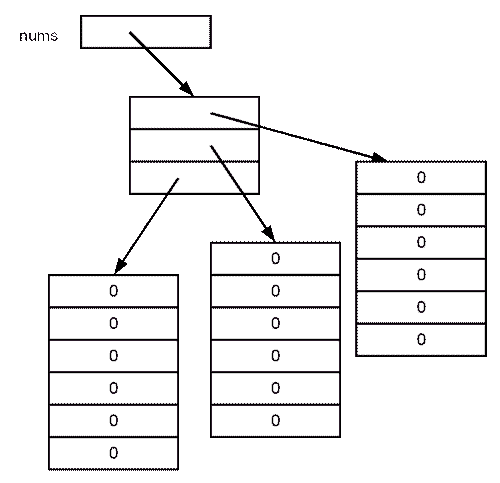Note that it is possible to replace any of the one-dimensional elements with a different one, or that the second-dimension arrays each have a different length - the following line would replace one of the arrays with another of a different length:

`nums = new int;`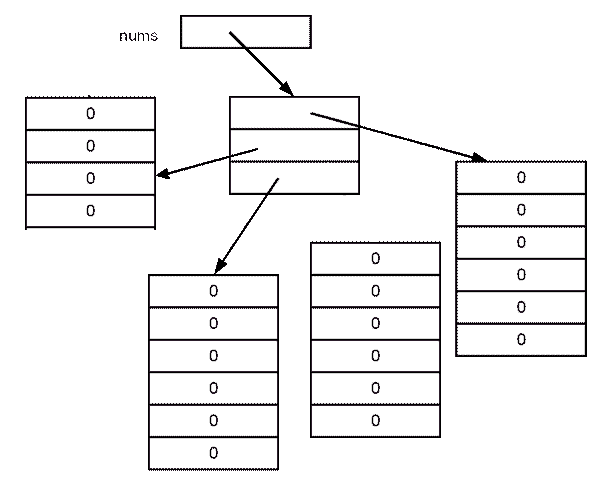## Example - Printing a Picture

This example uses a two-dimensional array preloaded with text characters that make up a picture

• There is a loop that processes each row (the first, or most significant, dimension of the array, each element of which is an array of characters).
• Within that loop, another loop prints each character without ending the line.
• Then, when the inner loop is done, a newline is printed.

## Code Sample:

Java-Arrays/Demos/ArrayPicture.java
```public class ArrayPicture {
public static void main(String[] args) {
char[][] imgData =
new char[][] {
{ ' ',' ',' ',' ',' ',' ',' ' },
{ ' ',' ',' ','0',' ',' ',' ' },
{ ' ',' ',' ','|',' ',' ',' ' },
{ ' ','0','-','+','-','0',' ' },
{ ' ',' ',' ','|',' ',' ',' ' },
{ ' ',' ',' ','0',' ',' ',' ' },
{ ' ',' ',' ',' ',' ',' ',' ' }
};

for (int row = 0; row < imgData.length ; row++ ) {
for (int col = 0; col < imgData[row].length; col++ ) {
System.out.print(imgData[row][col]);
}
System.out.println();
}
}
}```

### Code Explanation

Because multi-dimensional arrays are implemented as arrays of array references, it is possible to partially instantiate an array:

`int[][] nums = new int[];`

This creates `nums` as a two-dimensional array (better viewed in this case as an array of array references), and creates an array holding three `null` references to integer arrays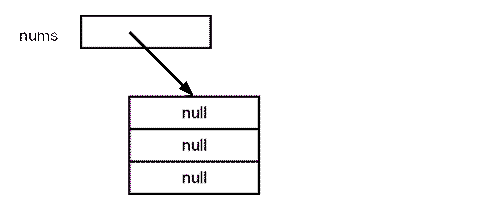## Typecasting with Arrays of Primitives

It is not possible to typecast an array of one type of primitive to an array of another type of primitive. For example, the following will cause compiler errors if the comment marks are removed:

## Code Sample:

Java-Arrays/Demos/ArrayTypecast.java
```// typecasts with arrays

public class ArrayTypecast {

public static void main(String[] args) {

int i = 5;
double d;

d = i;
i = (int) d;

int inums[] = { 1, 2, 3, 4, 5 };
double[] dnums;

// line below fails
//dnums = inums;

dnums = new double[] { 1.1, 2.2, 3.3, 4.4 };

// line below fails
//inums = (int[]) dnums;

}
}```

### Code Explanation

Neither an implicit or explicit typecast can be performed. With a single `int i`, the copy of it that is given to `d` can be expanded to a `double`. But, with the `int[] inums`, the value that would be given to `dnums` is just a copy of the reference to `inums`, so there is no way that each of the individual elements can be expanded to `double`.

The next chapter will discuss typecasting from arrays of one type of object to another.